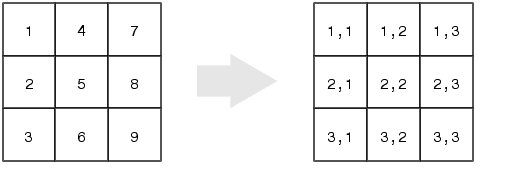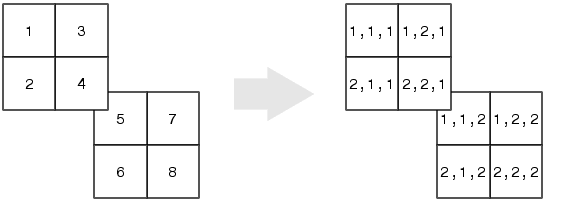MATLAB Function Referenceind2sub

Subscripts from linear index

Syntax

• ```[I,J] = ind2sub(siz,IND)
[I1,I2,I3,...,In] = ind2sub(siz,IND)
```

Description

The `ind2sub` command determines the equivalent subscript values corresponding to a single index into an array.

```[I,J] = ind2sub(siz,IND) ``` returns the matrices `I` and `J` containing the equivalent row and column subscripts corresponding to each linear index in the matrix `IND` for a matrix of size `siz`. `siz` is a 2-element vector, where `siz(1)` is the number of rows and `siz(2)` is the number of columns.

 Note    For matrices, `[I,J] = ind2sub(size(A),find(A>5))` returns the same values as `[I,J] = find(A>5)`.

```[I1,I2,I3,...,In] = ind2sub(siz,IND) ``` returns `n` subscript arrays `I1`,`I2`,`...`,`In` containing the equivalent multidimensional array subscripts equivalent to `IND` for an array of size `siz`. `siz` is an `n`-element vector that specifies the size of each array dimension.

Examples

Example 1. The mapping from linear indexes to subscript equivalents for a 3-by-3 matrix isThis code determines the row and column subscripts in a 3-by-3 matrix, of elements with linear indices 3, 4, 5, 6.

• ```IND = [3 4 5 6]
s = [3,3];
[I,J] = ind2sub(s,IND)

I =
3     1     2     3

J =
1     2     2     2
```

Example 2. The mapping from linear indexes to subscript equivalents for a 2-by-2-by-2 array isThis code determines the subscript equivalents in a 2-by-2-by-2 array, of elements whose linear indices 3, 4, 5, 6 are specified in the `IND` matrix.

• ```IND = [3 4;5 6];
s = [2,2,2];
[I,J,K] = ind2sub(s,IND)

I =
1     2
1     2

J =
2     2
1     1

K =
1     1
2     2
```

See Also

`find`, `size`, `sub2ind`

© 1994-2005 The MathWorks, Inc.